# Video Classification

The repository builds a quick and simple code for video classification (or action recognition) using UCF101 with PyTorch. A video is viewed as a 3D image or several continuous 2D images (Fig.1). Below are two simple neural nets models:

## Dataset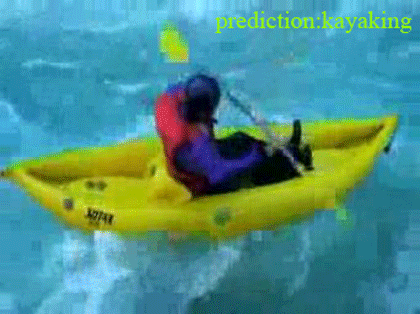UCF101 has total 13,320 videos from 101 actions. Videos have various time lengths (frames) and different 2d image size; the shortest is 28 frames.

To avoid painful video preprocessing like frame extraction and conversion such as OpenCV or FFmpeg, here I used a preprocessed dataset from feichtenhofer directly. If you want to convert or extract video frames from scratch, here are some nice tutorials:

## Models

### 1. 3D CNN (train from scratch)

Use several 3D kernels of size (a,b,c) and channels n, e.g., (a, b, c, n) = (3, 3, 3, 16) to convolve with video input, where videos are viewed as 3D images. Batch normalization and dropout are also used.

### 2. CNN + RNN (CRNN)

The CRNN model is a pair of CNN encoder and RNN decoder (see figure below):

• [encoder] A CNN function encodes (meaning compressing dimension) every 2D image x(t) into a 1D vector z(t) by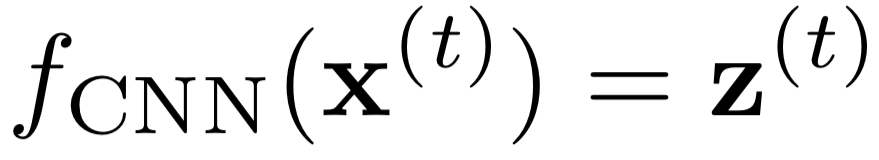• [decoder] A RNN receives a sequence input vectors z(t) from the CNN encoder and outputs another 1D sequence h(t). A final fully-connected neural net is concatenated at the end for categorical predictions.

• Here the decoder RNN uses a long short-term memory (LSTM) network and the CNN encoder can be:

1. trained from scratch
2. a pretrained model ResNet-152 using image dataset ILSVRC-2012-CLS.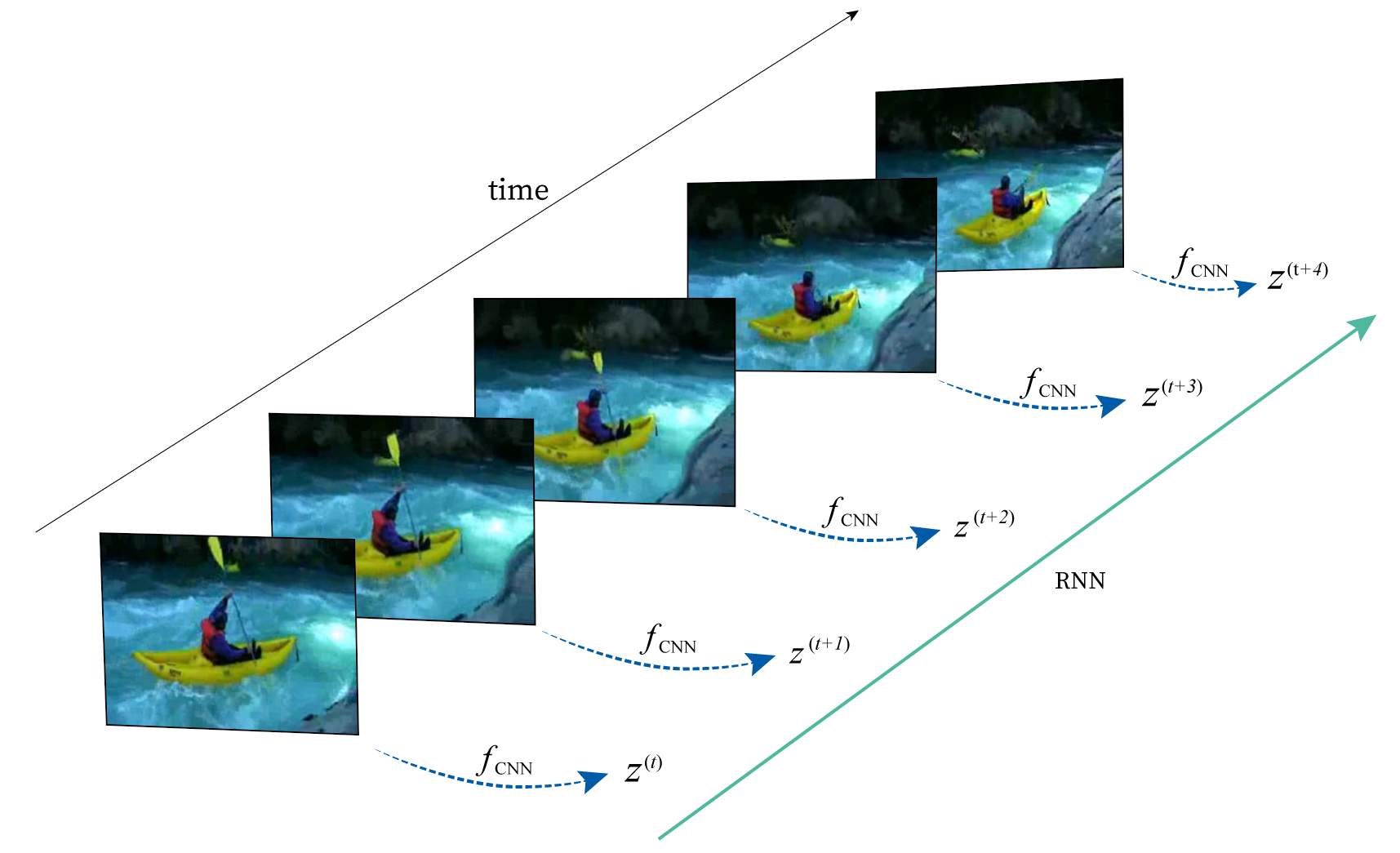## Training & testing

• For 3D CNN:

1. The videos are resized as (t-dim, channels, x-dim, y-dim) = (28, 3, 256, 342) since CNN requires a fixed-size input. The minimal frame number 28 is the consensus of all videos in UCF101.
2. Batch normalization, dropout are used.
• For CRNN, the videos are resized as (t-dim, channels, x-dim, y-dim) = (28, 3, 224, 224) since the ResNet-152 only receives RGB inputs of size (224, 224).

• Training videos = 9,990 vs. testing videos = 3,330

• In the test phase, the models are almost the same as the training phase, except that dropout has to be removed and batchnorm layer uses moving average and variance instead of mini-batch values. These are taken care by using "model.eval()".

## Usage

For tutorial purpose, I try to build code as simple as possible. Essentially, only 3 files are needed to for each model. eg., for 3D-CNN model

• `UCF101_3DCNN.py`: model parameters, training/testing process.
• `function.py`: modules of 3DCNN & CRNN, data loaders, and some useful functions.
• `UCF101actions.pkl`: 101 action names (labels), e.g, 'BenchPress', 'SkyDiving' , 'Bowling', etc.

### 0. Prerequisites

For convenience, we use preprocessed UCF101 dataset already sliced into RGB images feichtenhofer/twostreamfusion:

Put the 3 parts in same folder to unzip. The folder has default name: jpegs_256.

### 2. Set parameters & path

In `UCF101_CRNN.py`, for example set

``````data_path = "./UCF101/jpegs_256/"         # UCF101 video path
action_name_path = "./UCF101actions.pkl"
save_model_path = "./model_ckpt/"
``````

### 3. Train & test model

• For 3D CNN/ CRNN/ ResNetCRNN model, in each folder run
``````\$ python UCF101_3DCNN/CRNN/ResNetCRNN.py
``````

### 4. Model ouputs

By default, the model outputs:

• Training & testing loss/ accuracy: `epoch_train_loss/score.npy`, `epoch_test_loss/score.npy`

• Model parameters & optimizer: eg. `CRNN_epoch8.pth`, `CRNN_optimizer_epoch8.pth`. They can be used for retraining or pretrained purpose.

To check model prediction:

• Run `check_model_prediction.py` to load best training model and generate all 13,320 video prediction list in Pandas dataframe. File output: `UCF101_Conv3D_videos_prediction.pkl`.
• Run `check_video_predictions.ipynb` with Jupyter Notebook and you can see where the model gets wrong: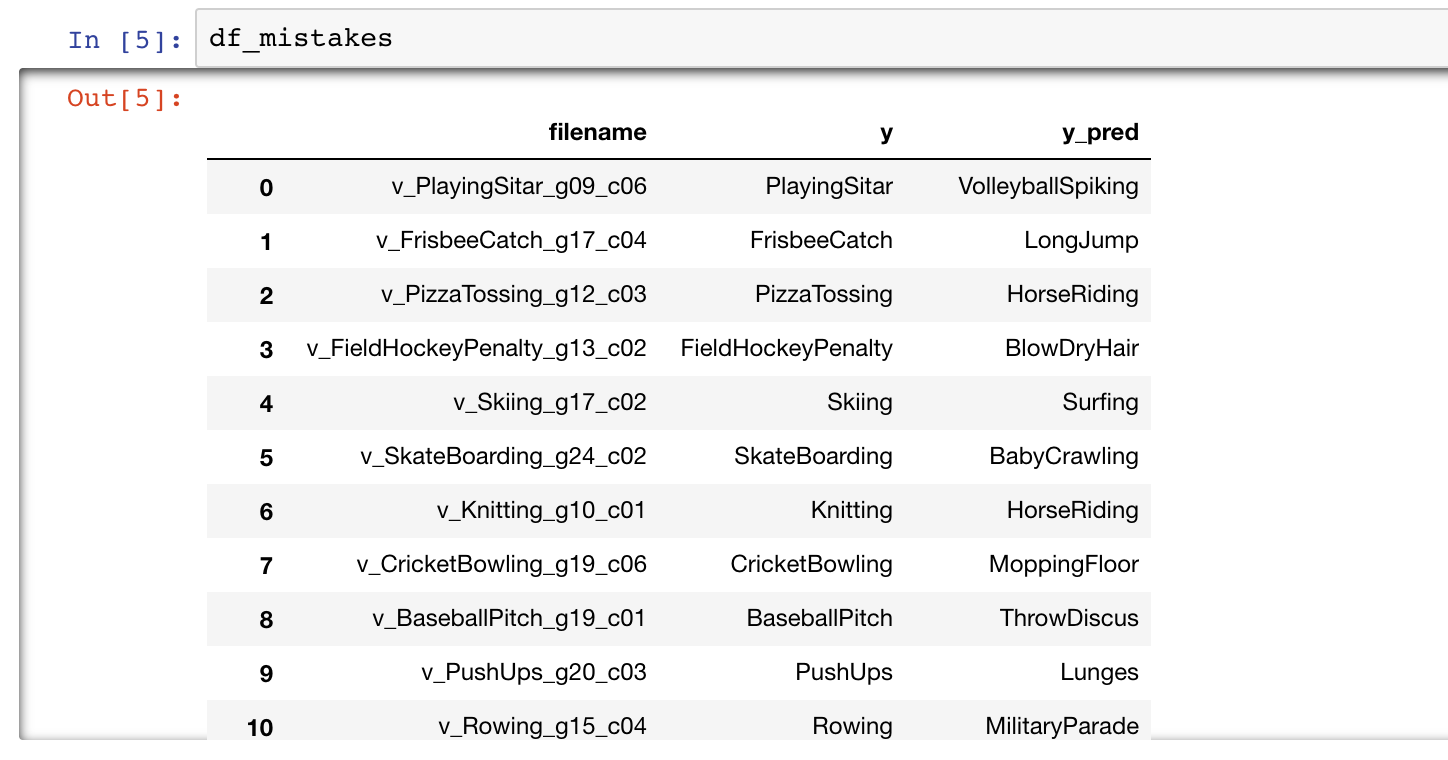## Version Warrning!

As of today (May 31, 2019), it is found that in Pytorch 1.1.0 flatten_parameters() doesn't work under torch.no_grad and DataParallel (for multiple GPUs). Early versions before Pytorch 1.0.1 still run OK. See Issues

Thanks to raghavgarg97's report.

## Device & performance

• The models detect and use multiple GPUs by themselves, where we implemented torch.nn.DataParallel.

• A field test using 2 GPUs (nVidia TITAN V, 12Gb mem) with my default model parameters and batch size `30~60`.

• Some pretrained models can be found here, thanks to the suggestion of MinLiAmoy.

network best epoch testing accuracy
3D CNN 4 50.84 %
2D CNN + LSTM 25 54.62 %
2D ResNet152-CNN + LSTM 53 85.68 %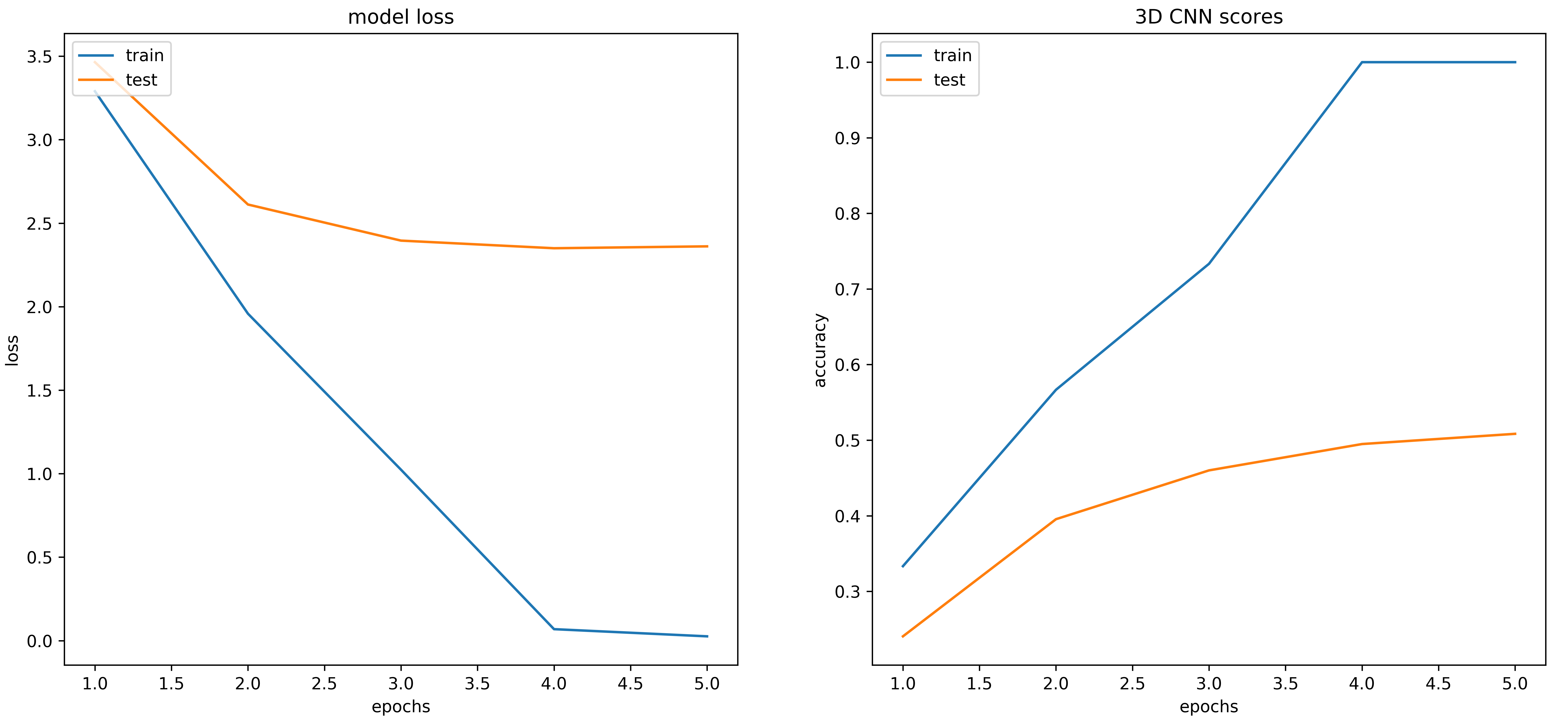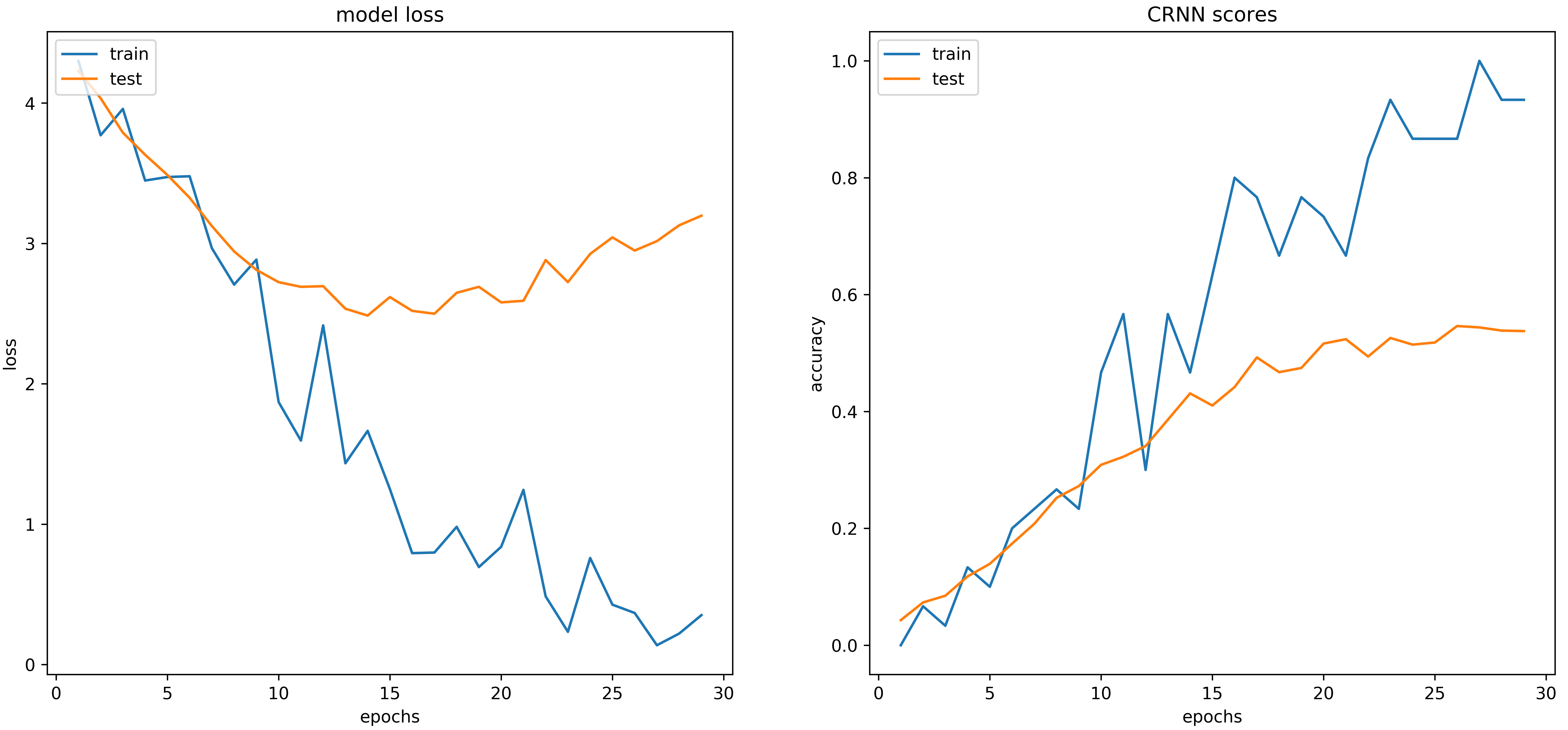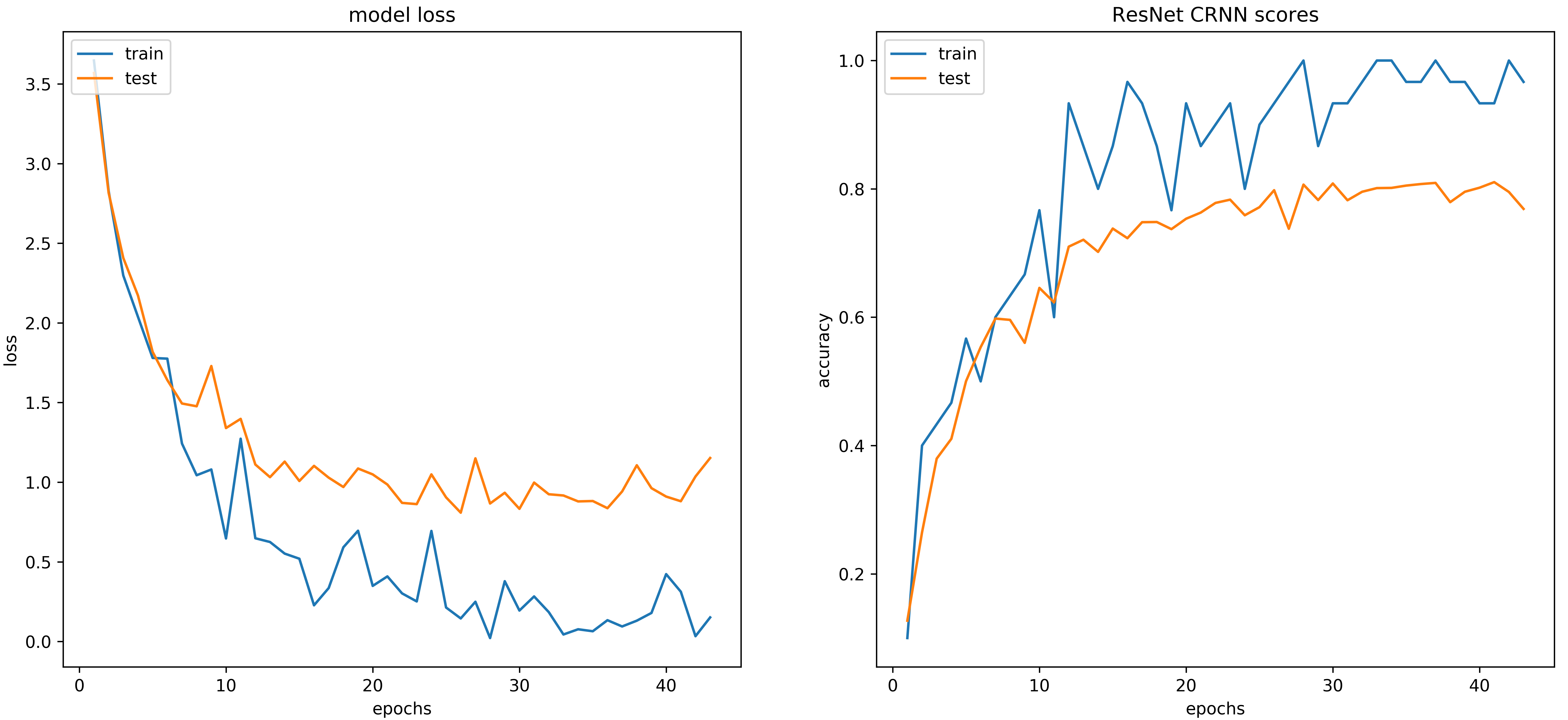Get A Weekly Email With Trending Projects For These Topics
No Spam. Unsubscribe easily at any time.
Jupyter Notebook (231,353
Cnn (3,128
Lstm (1,919
Rnn (1,178
Transfer Learning (998
Resnet (531
Pytorch Tutorial (275
Action Recognition (254
Related Projects